CyclePad
Design Library

# Design of a Diesel Cycle

## Goal

We look at the design of a Diesel cycle and at how its performance can be improved by changing its volumetric compression ratio. The Diesel cycle a closed cycle (where the system is a control mass), commonly used to model the cylinders of spark-ignition, internal combustion, automobile engines, i.e. gasoline engines.

## The General Idea

The Diesel cycle is very similar to the
Otto cycle in that both are closed cycles commonly used to model internal combustion engines. The difference between them is that the Diesel cycle is a compression-ignition cycle instead of a spark-ignition cycle like the Otto cycle. Compression-ignition cycles use fuels that begin combustion when they reach a temperature and pressure that occurs naturally at some point during the cycle and, therefore, do not require a separate energy source (e.g. from a spark plug) to burn. Diesel fuels are mixed so as to combust reliably at the proper thermal state so that Diesel cycle engines run well.

(We might note that most fuels will start combustion on their own at some temperature and pressure. But this is often not intended to occur and can result in the fuel combustion occurring too early in the cycle. For instance, when a gasoline engine - ordinarily an Otto cycle device - is run at overly high compression ratios, it can start "dieseling" where the fuel ignites before the spark is generated. It is often difficult to get such an engine to turn off since the usual method of simply depriving it of a spark may not work.

### Stages of Diesel Cycles

Diesel Cycles have four stages: compression, combustion, expansion, and cooling.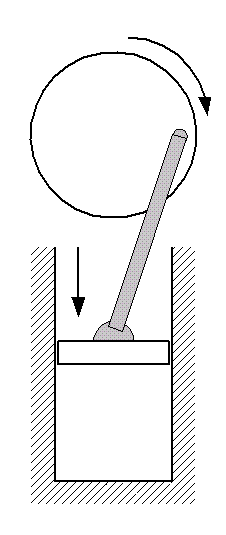Figure 3: moving down during compression

### Compression:

We start out with air at ambient conditions - often just outside air drawn into the engine. In preparation for adding heat to the air, we compress it by moving the piston down the cylinder. It is in this part of the cycle that we contribute work to the air. In the ideal Diesel cycle, this compression is considered to be isentropic.

It is at this stage that we set the volumetric compression ratio, r which is the ratio of the volume of the working fluid before the compression process to its volume after.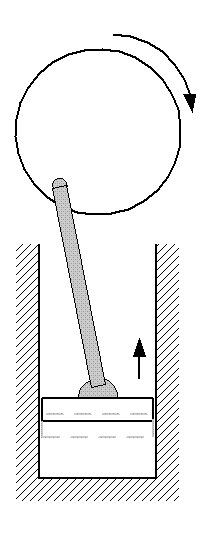Figure 4: starts at bottom dead center (shown dashed) during combustion

### Combustion:

Next, heat is added to the air by fuel combustion. This process begins just as the piston leaves its bottom dead center position. Because the piston is moving during this part of the cycle, we say that the heat addition is isochoric, like the cooling process.

Piston: starts at bottom dead center, begins moving up.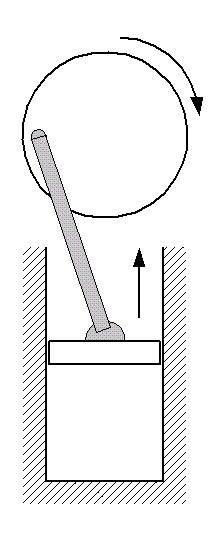Figure 1: moving up during expansion

### Expansion:

In the Diesel cycle, fuel is burned to heat compressed air and the hot gas expands forcing the piston to travel up in the cylinder. It is in this phase that the cycle contributes its useful work, rotating the automobile's crankshaft. We make the ideal assumption that this stage in an ideal Diesel cycle is isentropic.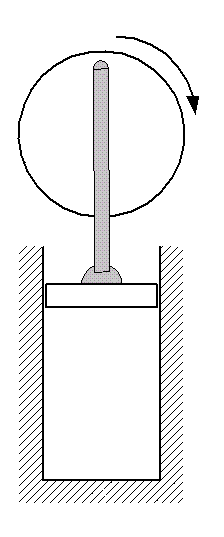Figure 2: top dead center during cooling

### Cooling:

Next, the expanded air is cooled down to ambient conditions. In an actual automobile engine, this corresponds to exhausting the air from the engine to the environment and replacing it with fresh air. Since this happens when the piston is at the top dead center position in the cycle and is not moving, we say this process is isochoric (no change in volume).

### P-v Diagram

The P-v diagram for a Diesel cycle is shown below.Figure 5: Diesel Cycle P-v diagram

## Example Diesel Cycle Design

### Problem Statement

For purposes of illustration, we will assume that we want to design a Diesel cycle that takes 1kg of air at ambient conditions of 15°C and 100kPa, compresses it to one-eighteenth its original volume and adds 1800kJ of heat to it in its combustion process. With what we know about Diesel cycles, that is all we need to completely describe the problem. (We also note that this is the same heat added during the Otto cycle design example.)

Below is a possible CyclePad design of an Diesel cycle.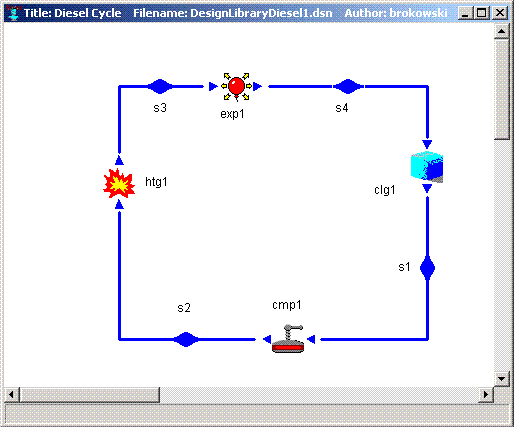Figure 6: Diesel Cycle in CyclePad

### the working fluid

We the most common working fluid for a Diesel cycle is air, since it is the cheapest thing in which to burn gasoline. We can choose air as our working fluid as air by selecting it as the substance in the meter window of any stuff.

## Description of Cycle Stages

We will briefly examine each statepoint and process of the Diesel cycle where design assumptions must be made, detailing each assumption. As we can see from the example design constraints, very few numbers need be specified to describe an ideal Diesel cycle. The rest of the assumptions are determined by applying background knowledge about the cycle. The principle numerical design decision is the compression ratio.

### Cycle Properties

Under the Cycle menu item, we can call up the Cycle Properties meter window. The only needed assumption here is that the cycle is a heat engine (a device to convert heat to work) so that CyclePad knows how to evaluate its efficiency.

### Pre-Compression (S1)

At this point, we have air entering the cylinder at ambient conditions, so we assume the temperature to be 15%deg;C and the pressure to be 100 kPa, as specified in the problem statement. This is also as good a point as any to specify the working fluid to be air and specify that the mass of the air is 1 kg.

### Compression Process (CMP1)

Here we assume both that the compression for out ideal Diesel cycle is isentropic and that our compression ratio is 18, as given in the problem statement.

### Post-Compression (S2)

No necessary specifications here.

### Combustion Process (HTG1)

Here we assume that the heating (which takes place just after the piston leaves the bottom dead center position) occurs with the piston moving under constant pressure, so it is isobaric. Here, we also assume the heat added (Q) to be 1800 kJ.

### Pre-Expansion (S3)

No necessary specifications here.

### Expansion Process (EXP1)

Since we are analyzing an ideal Diesel cycle, we assume that the expansion is isentropic. If we knew how much heat loss occurred in the expansion and the work it produced, we might be able to specify those here instead to model a non-ideal expansion process.

### Exhaust (Post-Expansion) (S4)

No necessary specifications here. This is where we release the used air to the environment.

### Cooling Process (CLG1)

Since the replacement of spent air with fresh air occurs when the piston is at its top dead center position, we assume the cooling process to be isochoric.

## Diesel Cycle Efficiency

We can look again in the Cycle Properties meter window to see that the thermal efficiency of the Diesel cycle we have built is about 59%.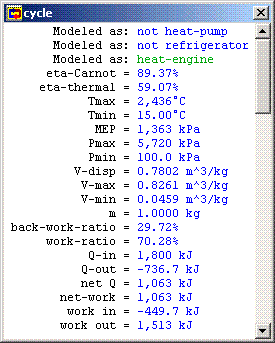Figure 7: Cycle Properties

One of our primary design specifications for the Diesel cycle was the volumetric compression ratio. We can use CyclePad's sensitivity tool to plot the cycle's thermal efficiency against this compression ratio.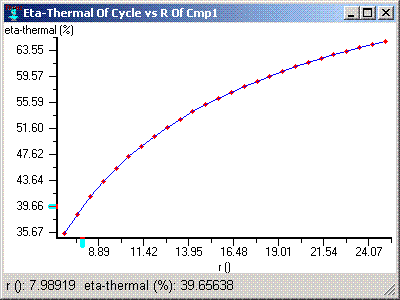Figure 8: Cycle Efficiency vs. Volumetric Compression Ratio

So, if we were to change the value of r to 25, our cycle efficiency would increase to 65%, which is a very large improvement.

We may recall from our Otto cycle design that increasing the compression ratio had the same beneficial effect on efficiency there as it does here for the Diesel cycle. In the Otto cycle, the limitation was that extremely high pressures developed in the cycle as we increase the compression ratio. In the Diesel cycle, however, we are not adding all our heat to the cycle while the piston is stuck at the position providing the smallest volume, so the extremely high pressures we saw in the Otto cycle do not develop as quickly. The figure below illustrates.Figure 9: Maximum Cycle Pressure vs. Volumetric Compression Ratio

Here, taking the compression ratio from 18 to 25, for instance, which takes our thermal efficiency to about 65%, only increases the maximum cycle pressure from under 5.7 MPa to just over 9 MPa. By comparison, the Otto cycle with a similar efficiency requires a compression ratio of about 13.5, which results in a maximum pressure of over 15 MPa.

## Sources

Whalley, P.B. 1992. Basic Engineering Thermodynamics. Oxford University Press. ISBN: 0-19-856255-1

Van Wylen, Sonntag, Borgnakke. 1994. Fundamentals of Classical Thermodynamics, 4th edition. John Wiley and Sons. ISBN: 0-471-59395-8

Contributed by: M. E. Brokowski
Initial Entry: 01/28/2002
Last Edited: 03/25/2002